### IMO Shortlist 2003 problem G2

Kvaliteta:
Avg: 2,5
Težina:
Avg: 6,0
Given three fixed pairwisely distinct points$A$,$B$,$C$ lying on one straight line in this order. Let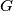$G$ be a circle passing through$A$ and$C$ whose center does not lie on the line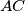$AC$. The tangents to$G$ at$A$ and$C$ intersect each other at a point$P$. The segment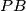$PB$ meets the circle$G$ at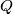$Q$.

Show that the point of intersection of the angle bisector of the angle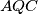$AQC$ with the line$AC$ does not depend on the choice of the circle$G$.
Izvor: Međunarodna matematička olimpijada, shortlist 2003